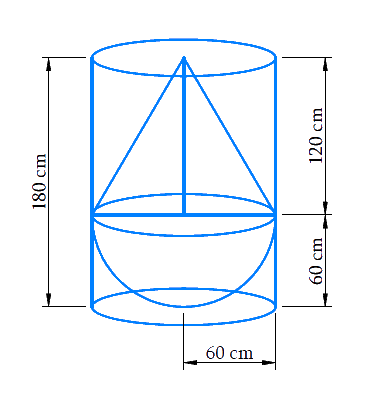# Ex.13.2 Q7 Surface Areas and Volumes Solution - NCERT Maths Class 10

Go back to  'Ex.13.2'

## Question

A solid consisting of a right circular cone of height $$120\,\rm {cm}$$ and radius $$60\rm\,{ cm}$$ standing on a hemisphere of radius $$60\rm\,{ cm}$$ is placed upright in a right circular cylinder full of water such that it touches the bottom. Find the volume of water left in the cylinder, if the radius of the cylinder is $$60\, \rm{cm}$$ and its height is $$180\,\rm {cm}.$$

## Text Solution

What is known?

A solid consisting of a right circular cone of height $$120\rm{cm}$$ and radius $$60\rm{cm}$$ standing on a hemisphere with the same radius is placed upright in a right circular cylinder of radius $$60\rm{cm}$$ and height $$180\rm{cm}$$, full of water such that it touches the bottom.

What is unknown?

Volume of water left in the cylinder.

Reasoning:

Draw a figure in accordance with the given information to visualize effectively.From the figure, we observe that the solid fits totally into the cylinder as the height of the solid is same as the height of the cylinder

Since the cylinder was full of water before immersing the solid into it, so the entirespace in the cylinder is occupied by the solid and the left-over water.

Volume of the cylinder $$=$$ volume of the solid $$+$$ volume of the water left in the cylinder

Therefore,

Volume of the water left in the cylinder $$=$$ volume of the cylinder $$–$$ volume of the solid

Hence,

Volume of the water left in the cylinder $$=$$ volume of the cylinder $$–$$ volume of the hemisphere $$–$$ volume of the cone

We will find the volume of the solid by using formulae;

Volume of the hemisphere\begin{align} = \frac{2}{3}\pi {r^3}\end{align}

where $$r$$ is the radius of the hemisphere

Volume of the cone\begin{align} = \frac{1}{3}\pi {r^2}h\end{align}

where $$r$$ and $$h$$ are the radius and height of the cone respectively.

Volume of the cylinder $$= \pi {r^2}h$$

where $$r$$ and $$h$$ are radius and height of the cylinder respectively.

Steps:

Radius of hemispherical part $$=$$ Radius of conical part $$=$$ Radius of cylinder$$= r = 60cm$$

Height of the conical part of solid $$= h = 120cm$$

Height of the cylinder, $$= H = 180cm$$

Volume of the water left in the cylinder $$=$$ volume of the cylinder $$–$$ volume of the hemisphere $$–$$ volume of the cone

\begin{align}&= \pi {r^2}H - \frac{1}{3}\pi {r^2}h - \frac{2}{3}\pi {r^3}\\&= \frac{1}{3}\pi {r^2}\left( {3H - h - 2r} \right)\\&= \frac{1}{3} \times \frac{{22}}{7} \times 60cm \times 60cm \times \left( {3 \times 180cm -120cm - 2 \times 60cm} \right)\\&= \frac{1}{3} \times \frac{{22}}{7} \times 60cm \times 60cm \times 300cm\\&= \frac{{7920000}}{7}c{m^3}\\&= 1131426.57c{m^3}\\&= \frac{{1131426.57}}{{1000000}}{m^3}\\&= 1.13142657{m^3}\end{align}

Learn from the best math teachers and top your exams

• Live one on one classroom and doubt clearing
• Practice worksheets in and after class for conceptual clarity
• Personalized curriculum to keep up with school